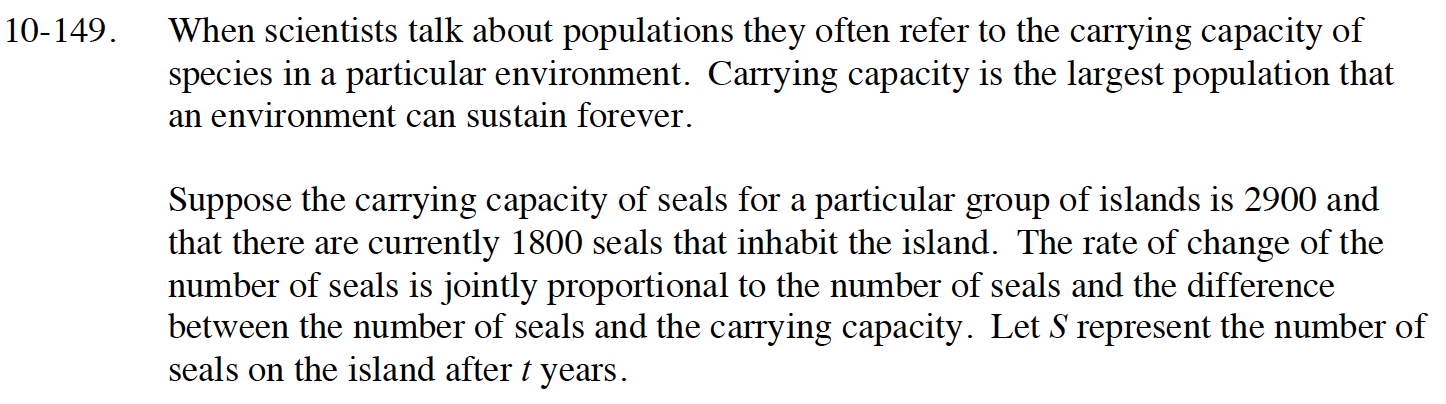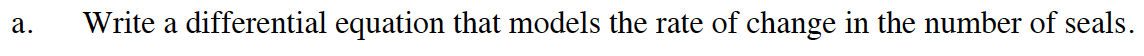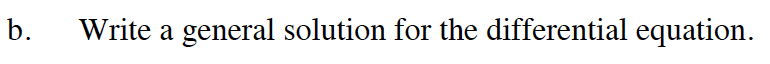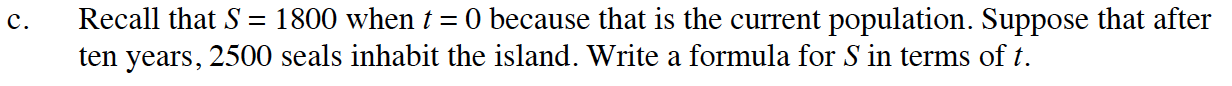### Home > CALC > Chapter 10 > Lesson 10.4.2 > Problem10-149

10-149.$\frac{dS}{dt}=kS(2900-S)$$\frac{dS}{S(2900-S)}=kdt$

$\frac{1}{S(2900-S)}=\frac{1/2900}{S}+\frac{1/2900}{2900-S}$

$\ln\Big|\frac{S}{2900-S}\Big|=2900kt+C$$S=\frac{2900\Big(\frac{18}{11}\Big)e^{2900kt}}{1+\frac{18}{11}e^{2900kt}}$